Lines, 2 Parallel Horizontal

2 parallel horizontal lines with arrows on both ends to show that they extend indefinitely.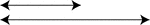Lines, 2 Parallel Horizontal

2 parallel horizontal lines with arrows on both ends to show that they extend indefinitely. The top…Lines, 2 Parallel Slanted

2 parallel lines with arrows on both ends to show that they extend indefinitely. The lines are slanting…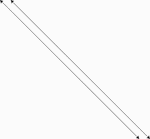Lines, 2 Parallel Slanted

2 parallel lines with arrows on both ends to show that they extend indefinitely. The lines are slanting…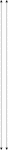Lines, 2 Parallel Vertical

2 parallel vertical lines with arrows on both ends to show that they extend indefinitely.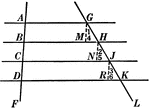4 Parallel Lines Cut By 2 Transversals

Illustration used to prove the theorem "If three or more parallel lines intercept equal segments on…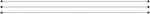Lines, 3 Parallel Horizontal

3 parallel horizontal lines with arrows on both ends to show that they extend indefinitely.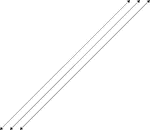Lines, 3 Parallel Slanted

3 parallel lines with arrows on both ends to show that they extend indefinitely. The lines are slanting…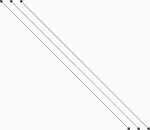Lines, 3 Parallel Slanted

3 parallel lines with arrows on both ends to show that they extend indefinitely. The lines are slanting…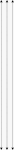Lines, 3 Parallel Vertical

3 parallel vertical lines with arrows on both ends to show that they extend indefinitely.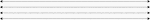Lines, 4 Parallel Horizontal

4 parallel horizontal lines with arrows on both ends to show that they extend indefinitely.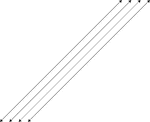Lines, 4 Parallel Slanted

4 parallel lines with arrows on both ends to show that they extend indefinitely. The lines are slanting…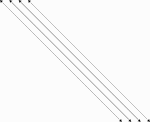Lines, 4 Parallel Slanted

4 parallel lines with arrows on both ends to show that they extend indefinitely. The lines are slanting…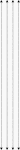Lines, 4 Parallel Vertical

4 parallel vertical lines with arrows on both ends to show that they extend indefinitely.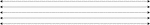Lines, 5 Parallel Horizontal

5 parallel horizontal lines with arrows on both ends to show that they extend indefinitely.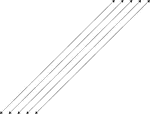Lines, 5 Parallel Slanted

5 parallel lines with arrows on both ends to show that they extend indefinitely. The lines are slanting…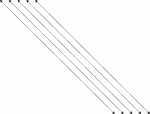Lines, 5 Parallel Slanted

5 parallel lines with arrows on both ends to show that they extend indefinitely. The lines are slanting…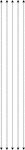Lines, 5 Parallel Vertical

5 parallel vertical lines with arrows on both ends to show that they extend indefinitely.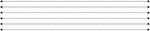Lines, 6 Parallel Horizontal

6 parallel horizontal lines with arrows on both ends to show that they extend indefinitely.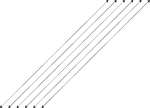Lines, 6 Parallel Slanted

6 parallel lines with arrows on both ends to show that they extend indefinitely. The lines are slanting…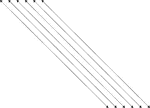Lines, 6 Parallel Slanted

6 parallel lines with arrows on both ends to show that they extend indefinitely. The lines are slanting…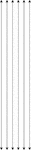Lines, 6 Parallel Vertical

6 parallel vertical lines with arrows on both ends to show that they extend indefinitely.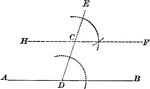Construction of a Straight Line Parallel to a Given Straight Line

Illustration of the construction used to create straight line parallel to a given straight line through…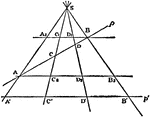Cross-Ratio Four Points

The cross-ratio of four points in a line is equal to the cross-ratio of their projections on any other…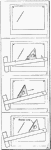Drawing Parallel Lines Exercise

Parallel lines are drawn by using a triangle in combination with a T-square. To draw a line parallel…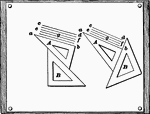Drawing Parallel Lines

Using a drawing board and pairs of triangles to draw parallel lines.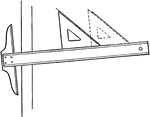Drawing Parallel Lines Using T-square and Triangle

Parallel lines are drawn by holding the triangle against the T-square at an angle, or another triangle,…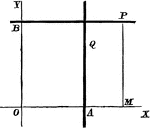Straight Line

Equation of a straight line: a) parallel to y-axis, b) parallel to x-axis.Parallel Lines Cut by Transversals

Illustration that shows if two parallel lines are cut by three or more transversals that pass through…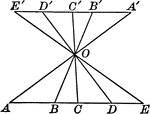Parallel Lines Cut by Transversals

Illustration that shows if two parallel lines are cut by three or more transversals that pass through…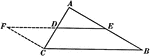Line Joining Midpoints on a Triangle

Illustration used to prove "The line joining the mid-points of two sides of a triangle is parallel to…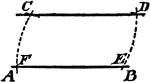Construction Of A Parallel

An illustration showing the construction used to erect a parallel line. "With C as a center, draw the…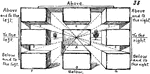Positioning

"Observe: That when drawn above the level of the eye, the bottom faces can be seen. (Boxes H, C and…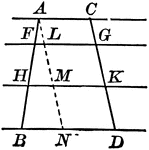Parallel Lines Cut by Parallels, Corresponding Intercepts Proportional

Illustration to show that if parallel lines are cut by any number of parallels, the corresponding intercepts…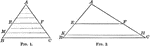Triangle Divided by Parallel Lines to Form Proportionate Triangles

Illustration to show that if a line is drawn through two sides of a triangle parallel to the third side,…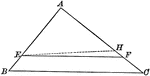Triangle Divided by Parallel Lines to Form Proportionate Triangles

Illustration to show that if a line divides two sides of a triangle proportionally, it is parallel to…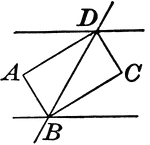Rectangle Formed When Two Parallel Lines are Cut by a Transversal

Illustrations to show that if two parallel lines are cut by a transversal, the bisectors of the interior…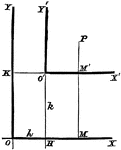Axes Shift

Any system of coordinate axes to another set which is parallel to the former, but with different origins.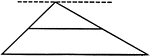Line Bisecting Side of a Triangle

Illustration used to prove "The line bisecting one side of a triangle and parallel to another side bisects…Sphere With 8-inch Diameter Cut by Planes

An 8-inch sphere cut by parallel planes, one 2 inches from center and the other 6 inches from center.…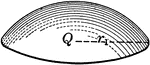Zones or Segments of Spheres

An illustration of a zone of a sphere. A zone occurs when a sphere is cut by parallel planes that are…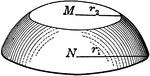Zones or Segments of Spheres

An illustration of a zone of a sphere. A zone occurs when a sphere is cut by parallel planes that are…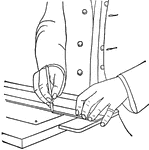T Square

A person using a T square to draw straight lines.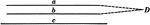Parallel Lines Theorem

Illustration used to prove that "If two straight lines are parallel to a third straight line, they are…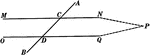Parallel Lines Cut By A Transversal Theorem

Illustration used to prove the theorem, "If two straight lines are cut by a transversal making a pair…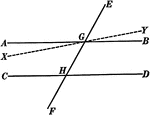Parallel Lines Cut By A Transversal Theorem

Illustration used to prove the theorem, "If two parallel lines are cut by a transversal, the alternate…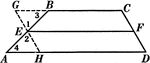Line Bisecting Trapezoid Sides

Illustration used to prove the theorem "The line bisecting one of the non parallel sides of a trapezoid…